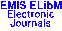PUBLICATIONS DE L'INSTITUT MATHÉMATIQUE (BEOGRAD) (N.S.) Vol. 70(84), pp. 63--68 (2001)

Previous Article

Next Article

Contents of this Issue

Other Issues

ELibM Journals

ELibM Home

EMIS Home

## ESTIMATING OF PARAMETERS: NUAR(1) PROCESS

### Miroslav M. Risti\'c and Biljana \v C. Popovi\'c

Prirodno-matemati\v cki fakultet, Ni\v s, Yugoslavia

Abstract: We applied the method of conditional least squares for estimating parameters of NUAR(1). This process can be represented as the random coefficient autoregressive time series of the form $$X_n=U_n X_{n-1}+V_n,$$ where $\{(U_n,V_n)\}$ is the sequence of independent identically distributed random vectors such that supply the elements of the sequence $\{X_n\}$ with $\mathcal{U}(0,1)$ marginal distribution. Defined estimates were the functions of the estimates of moments $E(U_n)$ and $E(U_n V_n)$ and they are strong consistent and asymptotically normally distributed.

Keywords: conditional least squares estimation; uniform autoregressive process; asymptotic normality; strong consistency

Classification (MSC2000): 62M10

Full text of the article: